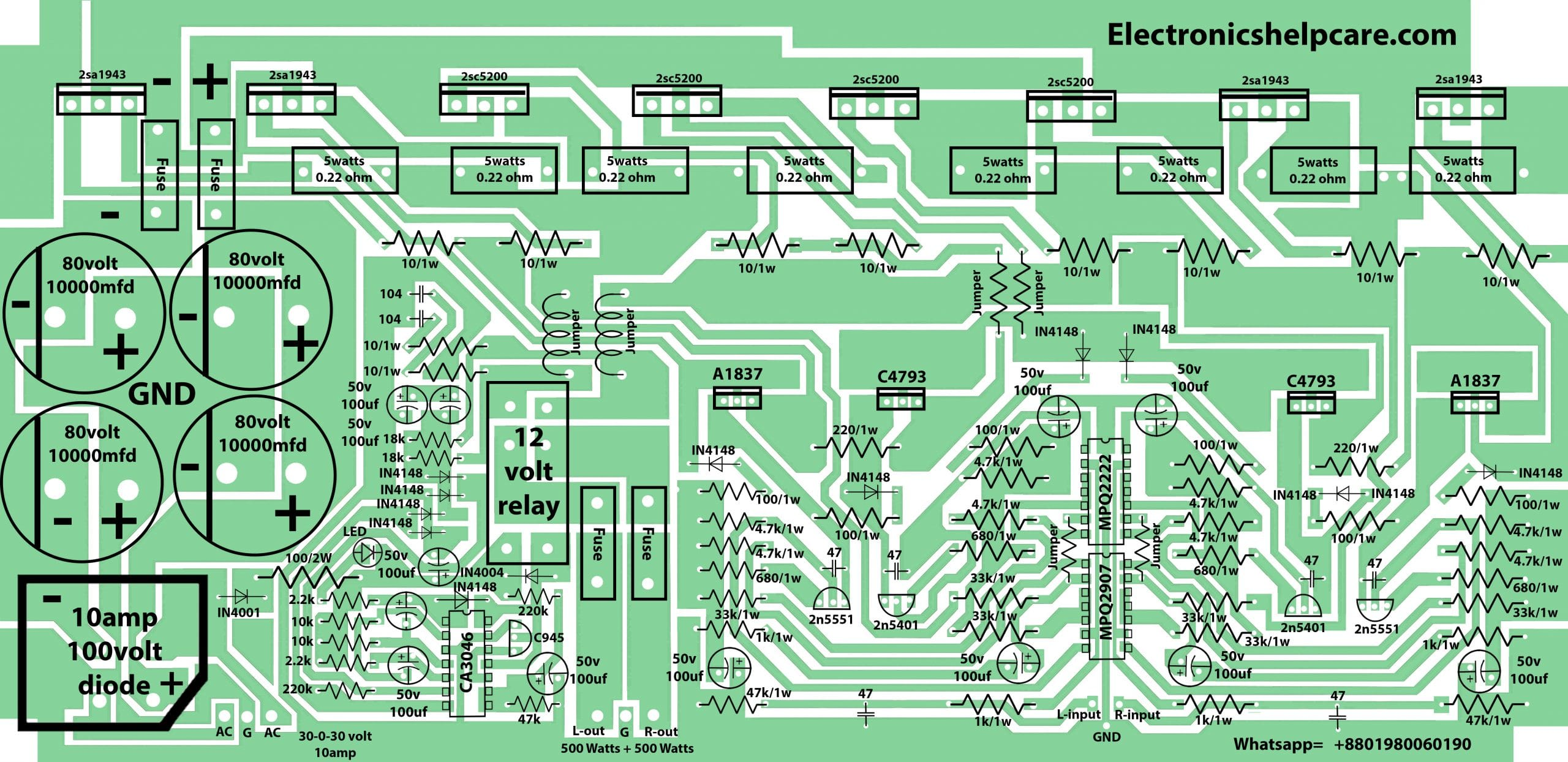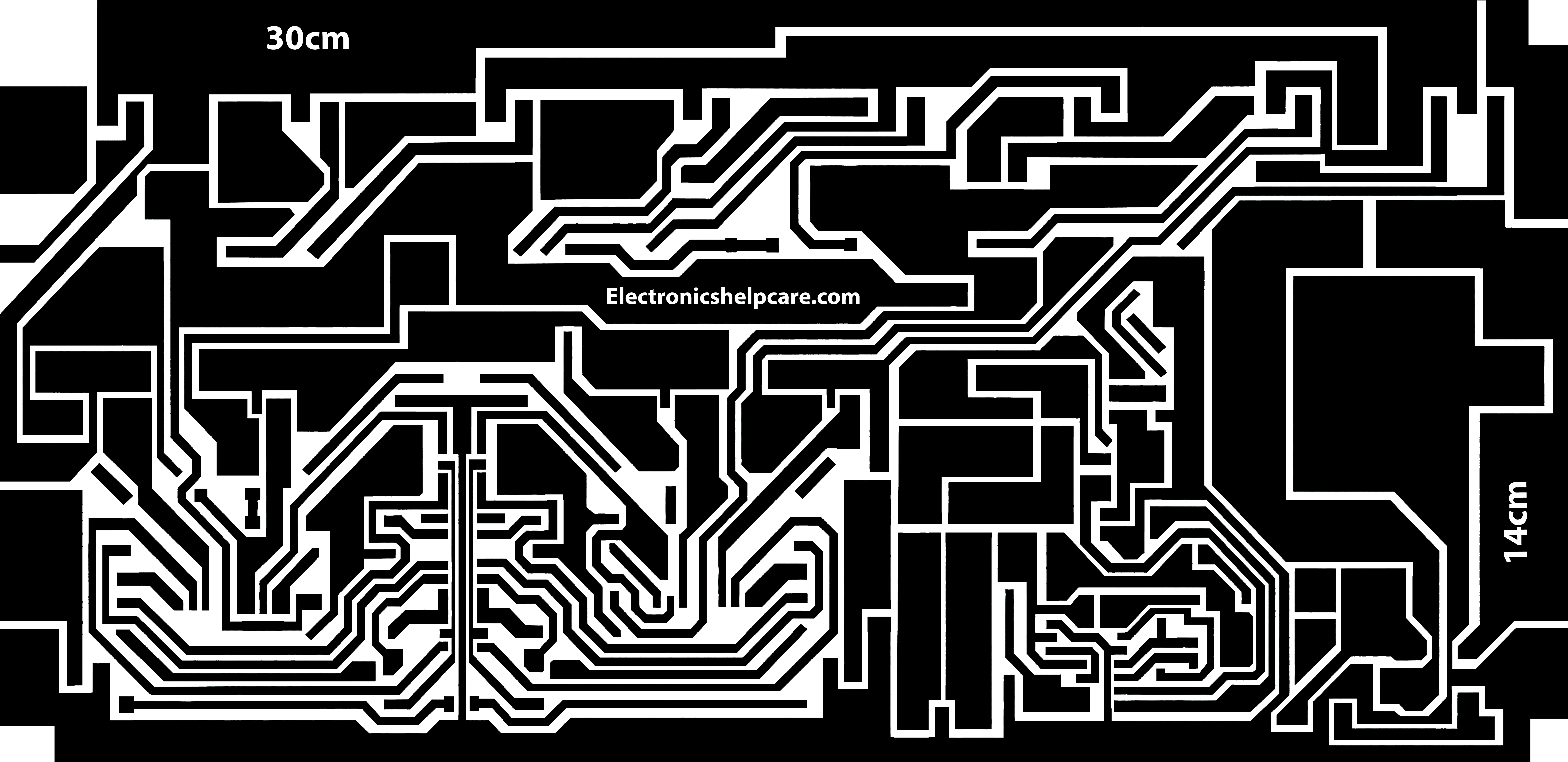Breaking News

# 1000 watts amplifier circuit diagram using 2sc5200 and 2sa1943

## 1000 watts amplifier circuit diagram using 2sc5200 and 2sa1943

This is 1000 watts amplifier circuit diagram using 2sc5200 and 2sa1943. this circuit has speaker short circuit protection. This circuit is a stereo amplifier. it’s a PNP and NPN amplifier. 2sc5200 is an NPN transistor and 2sa1943 is the PNP transistor. we can use another PNP and NPN transistor also. one transistor can take 1.3 amperes. here we can use 30-0-30 voltage and 10 amperes. 0 to 30 positive and 0 to 30 negative voltage. 0 to positive is 0 to 30 volt 10 amperes. another 0 to negative is 0 to 30 volt 10 amperes. So, here we count 30 voltage and 10+10=20amperes.

We know that voltage X amperes= Watts. so, our voltage is 30 and amperes is 20. 30X20=600 watts we can get. here in this video, we can watch that we use 25-0-25 voltage and 10 amperes.

This is the diagram for this amplifier.1000 watts amplifier circuit diagram using 2sc5200 and 2sa1943

If you need help please WhatsApp us.2sc5200 and 2sa1943

If you need any other circuit diagram please visit us

www.electronicshelpcare.com

### About Electronics Help CareRamen Bairagee

## Speaker protection circuit diagram

Speaker protection circuit diagram.: This is the Speaker protection circuit diagram. It can protect our …